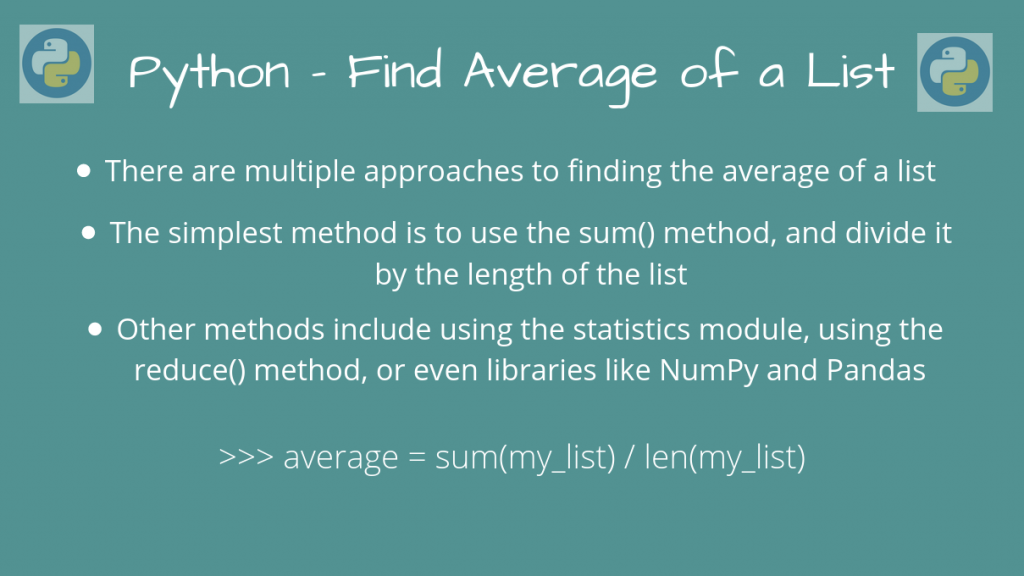# Find the Average of a List in Python with 5 Easy MethodsThere are many ways to find the average of a list in Python, provided all of them are of the same type. In this article, we’ll look at some of the methods to find the average element of a Python List.

Let’s get started!

## Method 1: Use reduce() to find the average of a list in Python

We can use the reduce() method, along with a lambda function (As shown here).

We’ll sum up the elements using the lambda, and divide the result by the length of the list since average = (Sum of all elements) / (Number of elements)

So, the corresponding expression is:

```average = (reduce(lambda x, y: x + y, input_list)) / len(input_list)
```

Let’s take a full example, to show this in action.

```from functools import reduce

input_list = [3, 2, 1, 5, 7, 8]

average = (reduce(lambda x, y: x + y, input_list)) / len(input_list)

print("Average of List:", average)
```

Output

```Average of List: 4.333333333333333
```

This is indeed the correct output, since the average is: (3 + 2 + 1 + 5 + 7 + 8) / 6 = 26 / 6 = 4.333

We cannot pass elements of different types, since the `+` operand may not work. So, all the elements of the list must be type compatible with the `+` operand in order for this to work. This is one of the methods we can find the average of a list in Python.

## Method 2: Using the sum() method (Recommended)

Instead of using the `reduce()` method, we can directly find the sum using `sum(list)`, and divide it by the length.

```input_list = [3, 2, 1, 5, 7, 8]

average = sum(input_list) / len(input_list)

print("Average of List:", average)
```

We’ll get the same output as before!

This is the recommended method of finding the average of a list in Python, since it uses only built-in functions, and is really simple to use.

## Method 3: Using statistics.mean()

For Python versions of 3.4 and above, we can also use the `statistics` module to find the average of a list in Python.

```import statistics

input_list = [3, 2, 1, 5, 7, 8]

average = statistics.mean(input_list)

print("Average of List:", average)
```

## Method 4: Using numpy.mean()

If you want to use NumPy based methods, `numpy.mean()` will do this for you.

```import numpy as np

input_list = [3, 2, 1, 5, 7, 8]

average = np.mean(input_list)

print("Average of List:", average)
```

You can also convert the list into a numpy array, and then get the mean using `ndarray.mean`. You can use whichever is better for your use-case, but they perform about the same.

```import numpy as np

input_list = [3, 2, 1, 5, 7, 8]

# Convert to a numpy ndarray and get the mean
# using ndarray.mean
average = np.array(input_list).mean

print("Average of List:", average)
```

## Method 5: Using pandas Series.mean()

If you’re using `Pandas`, you can get the mean of a list using `pandas.Series(list).mean()`

```import pandas as pd

input_list = [3, 2, 1, 5, 7, 8]

# Convert the list to a Pandas Series using pd.Series(list)
# and then get it's mean using series.mean()
average = pd.Series(input_list).mean()

print("Average of List:", average)
```

Output

```Average of List: 4.333333333333333
```

## Conclusion

In this article, we learned how we could find the average of a list in Python, using different approaches.